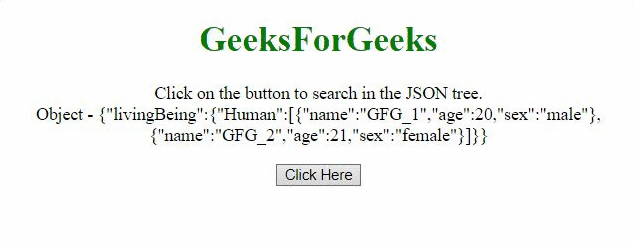# How to search JSON tree with the help of jQuery ?

Given a JSON Object’s tree and the task is to find a particular piece of information. Suppose we want to find the information about a particular person in that object then how do we do that. Here are two approaches that are discussed below:

Approach 1: In this approach, we will use each() method and pass the real array of objects to the method by using the dot operator and then in the anonymous function find the person by comparing its name.

Example:

## HTML

 ` ` `<``html``> ` ` `  `<``head``> ` `    ``<``script` `src``= ` `"https://code.jquery.com/jquery-3.5.0.js"``> ` `    `` ` ` ` ` `  `<``body` `style``=``"text-align:center;"``> ` ` `  `    ``<``h1` `style``=``"color: green"``> ` `        ``GeeksForGeeks ` `    `` ` `     `  `    ``<``p` `id``=``"GFG_UP"``> ` ` `  `    ``<``button` `onclick``=``"gfg_Run()"``> ` `        ``Click Here ` `    `` ` `     `  `    ``<``p` `id``=``"GFG_DOWN"``> ` ` `  `    ``<``script``> ` `        ``var elUp = document.getElementById("GFG_UP"); ` `        ``var elDown = document.getElementById("GFG_DOWN"); ` ` `  `        ``var GFG_Object = { ` `            ``"livingBeing": { ` `                ``"Human": [ ` `                    ``{ ` `                        ``"name": "GFG_1", ` `                        ``"age": 20, ` `                        ``"sex": "male" ` `                    ``}, { ` `                        ``"name": "GFG_2", ` `                        ``"age": 21, ` `                        ``"sex": "female" ` `                    ``} ` `                ``] ` `            ``} ` `        ``}; ` ` `  `        ``elUp.innerHTML = ` `            ``"Click on the button to search in" ` `            ``+ " the JSON tree.<``br``>Object - " ` `            ``+ JSON.stringify(GFG_Object); ` ` `  `        ``function gfg_Run() { ` `            ``\$.each(GFG_Object.livingBeing.Human,  ` `                ``function (i, v) { ` `                 `  `                ``if (v.name == "GFG_1") { ` `                    ``elDown.innerHTML =  ` `                        ``"The age of person('GFG_1') is  " ` `                        ``+ v.age; ` `                    ``return; ` `                ``} ` `            ``}); ` `        ``}    ` `    `` ` ` ` ` `  ` `

Output:Approach 2: In this approach, we will use grep() method and pass the actual array of objects to the method by using dot operator and then return the object which matches the criteria and then we print the age of the person.

Example:

## HTML

 ` ` `<``html``> ` ` `  `<``head``> ` `    ``<``script` `src``= ` `"https://code.jquery.com/jquery-3.5.0.js"``> ` `    `` ` ` ` ` `  `<``body` `style``=``"text-align:center;"``> ` ` `  `    ``<``h1` `style``=``"color: green"``> ` `        ``GeeksForGeeks ` `    `` ` `     `  `    ``<``p` `id``=``"GFG_UP"``> ` `     `  `    ``<``button` `onclick``=``"gfg_Run()"``> ` `        ``Click Here ` `    `` ` `     `  `    ``<``p` `id``=``"GFG_DOWN"``> ` `     `  `    ``<``script``> ` `        ``var elUp = document.getElementById("GFG_UP"); ` `        ``var elDown = document.getElementById("GFG_DOWN"); ` `        ``var GFG_Object = { ` `            ``"livingBeing": { ` `                ``"Human": [ ` `                    ``{ ` `                        ``"name": "GFG_1", ` `                        ``"age": 20, ` `                        ``"sex": "male" ` `                    ``}, { ` `                        ``"name": "GFG_2", ` `                        ``"age": 21, ` `                        ``"sex": "female" ` `                    ``} ` `                ``] ` `            ``} ` `        ``}; ` `        ``elUp.innerHTML ` `            ``= "Click on the button to search in" ` `            ``+ " the JSON tree.<``br``>Object - " ` `            ``+ JSON.stringify(GFG_Object); ` ` `  `        ``function gfg_Run() { ` `            ``var res ` `                ``= \$.grep(GFG_Object.livingBeing.Human,  ` `                ``function (element, index) { ` `                    ``return (element.name === 'GFG_1'); ` `                ``}); ` `            ``elDown.innerHTML =  ` `                ``"The age of person('GFG_1') is "  ` `                ``+ res.age; ` `        ``}    ` `    `` ` ` ` ` `  ` `

Output:My Personal Notes arrow_drop_upCheck out this Author's contributed articles.

If you like GeeksforGeeks and would like to contribute, you can also write an article using contribute.geeksforgeeks.org or mail your article to contribute@geeksforgeeks.org. See your article appearing on the GeeksforGeeks main page and help other Geeks.

Please Improve this article if you find anything incorrect by clicking on the "Improve Article" button below.

Practice Tags :

Be the First to upvote.

Please write to us at contribute@geeksforgeeks.org to report any issue with the above content.